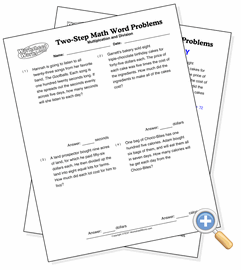# Two-Step Word Problems

## Multiplication, then DivisionSolve word problems that require two separate operations

Two-step word problems have three numbers which must be operated on separately, and in the right order. They take much more understanding of the problem than simple word problems because they provide the information in a less structured form. For these problems, finding the answer involves multiplying two numbers and then dividing the product by another number.